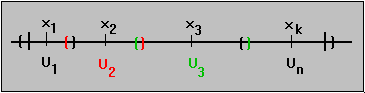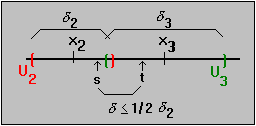#Interactive Real Analysis

Next | Previous | Glossary | Map

## 6.2. Continuous Functions

### Theorem 6.2.9: Continuity and Uniform Continuity

If f is uniformly continuous in a domain D, then f is continuous in D.

If f is continuous on a compact domain D, then f is uniformly continuous in D.

### Proof:

The first part of the proof is obvious. If |f(t) - f(s)| is small whenever |s - t| is small, regardless of the particular location of s and t, then in particular |f(x) - f( x0)| must be small when |x - x0| is small.

The second part is much more complicated, and relies on the structure of compact sets on the real line. Recall that a set is compact if every open cover has a finite subcover. We first want to define a suitable open cover: pick an> 0. For every fixed x0 in the compact set D there exists a (possibly different)(x0) > 0 such that

| f( x0) - f(x) | <if | x0- x | < 1/2(x0)

wheredepends on x0. Define

U(x0) = { x: |x0 - x| < 1/2(x0)} and U = { U(x0): x0 is contained in D}

Then U is an open cover of D, so by compactness can be reduced to a finite subcover. Suppose that the sets U1, U2, ..., Un cover the set D:Let= 1/2 min{(x1),(x2), ...(xn)}

Since this is a minimum over a finite set, we know that> 0. Now take any two numbers t, s in D such that |t - s| <. Since the finite collection covers the compact set D we know that s is contained in, say, U2. How far away from the center of U2 is t then ?By the choice ofwe know that

|t - x2| < |t - s| + |s - x2| <+ 1/2(x2) < 1/2 (( x2) +( x2)) =( x2)

Now we are almost done, because if |t - s| <then

|f(t) - f(s)| < |f(t) - f( x2)| + |f( x2) - f(s)|

The first difference on the right is less than epsilon because |t - x2| <(x2). The second one is also less than epsilon because s is contained in the set U2. Hence, the difference on the left is less than twice epsilon. But now it should be easy for you to modify the proof so that we can arrive at

|f(t) - f(s)| <whenever |t - s| <in D

That finishes the proof.Next | Previous | Glossary | Map